Download Presentation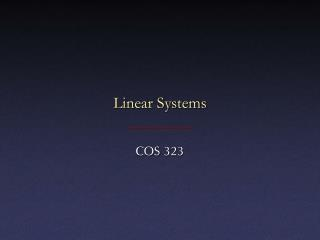Linear Systems

# Linear Systems - PowerPoint PPT Presentation

Linear Systems. COS 323. Linear Systems. Linear Systems. Solve Ax=b, where A is an n  n matrix and b is an n  1 column vector Can also talk about non-square systems where A is m  n , b is m  1, and x is n  1 Overdetermined if m > n : “more equations than unknowns”I am the owner, or an agent authorized to act on behalf of the owner, of the copyrighted work described.
Download Presentation## Linear Systems

An Image/Link below is provided (as is) to download presentation

Download Policy: Content on the Website is provided to you AS IS for your information and personal use and may not be sold / licensed / shared on other websites without getting consent from its author.While downloading, if for some reason you are not able to download a presentation, the publisher may have deleted the file from their server.

- - - - - - - - - - - - - - - - - - - - - - - - - - E N D - - - - - - - - - - - - - - - - - - - - - - - - - -
Presentation Transcript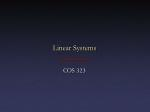### Linear Systems

COS 323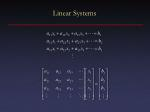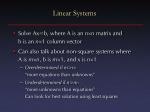Linear Systems
• Solve Ax=b, where A is an nn matrix andb is an n1 column vector
• Can also talk about non-square systems whereA is mn, b is m1, and x is n1
• Overdetermined if m>n:“more equations than unknowns”
• Underdetermined if n>m:“more unknowns than equations”Can look for best solution using least squares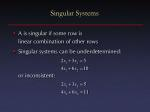Singular Systems
• A is singular if some row islinear combination of other rows
• Singular systems can be underdetermined:or inconsistent: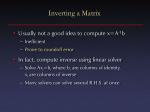Inverting a Matrix
• Usually not a good idea to compute x=A-1b
• Inefficient
• Prone to roundoff error
• In fact, compute inverse using linear solver
• Solve Axi=bi where bi are columns of identity,xi are columns of inverse
• Many solvers can solve several R.H.S. at once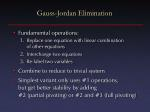Gauss-Jordan Elimination
• Fundamental operations:
• Replace one equation with linear combinationof other equations
• Interchange two equations
• Re-label two variables
• Combine to reduce to trivial system
• Simplest variant only uses #1 operations,but get better stability by adding#2 (partial pivoting) or #2 and #3 (full pivoting)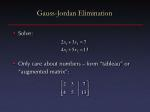Gauss-Jordan Elimination
• Solve:
• Only care about numbers – form “tableau” or “augmented matrix”: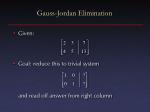Gauss-Jordan Elimination
• Given:
• Goal: reduce this to trivial systemand read off answer from right column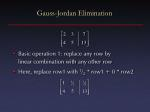Gauss-Jordan Elimination
• Basic operation 1: replace any row bylinear combination with any other row
• Here, replace row1 with 1/2 * row1 + 0 * row2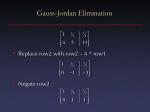Gauss-Jordan Elimination
• Replace row2 with row2 – 4 * row1
• Negate row2Gauss-Jordan Elimination
• Replace row1 with row1 – 3/2 * row2
• Read off solution: x1 = 2, x2 = 1Gauss-Jordan Elimination
• For each row i:
• Multiply row i by 1/aii
• For each other row j:
• Add –aji times row i to row j
• At the end, left part of matrix is identity,answer in right part
• Can solve any number of R.H.S. simultaneouslyPivoting
• Consider this system:
• Immediately run into problem:algorithm wants us to divide by zero!
• More subtle version:Pivoting
• Conclusion: small diagonal elements bad
• Remedy: swap in larger element from somewhere elsePartial Pivoting
• Swap rows 1 and 2:
• Now continue:Full Pivoting
• Swap largest element onto diagonal by swapping rows 1 and 2 and columns 1 and 2:
• Critical: when swapping columns, must remember to swap results!Full Pivoting
• Full pivoting more stable, but only slightly

* Swap results 1 and 2Operation Count
• For one R.H.S., how many operations?
• For each of n rows:
• Do n times:
• For each of n+1 columns:
• One add, one multiply
• Total = n3+n2 multiplies, same # of adds
• Asymptotic behavior: when n is large, dominated by n3Faster Algorithms
• Our goal is an algorithm that does this in1/3n3 operations, and does not requireall R.H.S. to be known at beginning
• Before we see that, let’s look at a fewspecial cases that are even fasterTridiagonal Systems
• Common special case:
• Only main diagonal + 1 above and 1 belowSolving Tridiagonal Systems
• When solving using Gauss-Jordan:
• Constant # of multiplies/adds in each row
• Each row only affects 2 othersRunning Time
• 2n loops, 4 multiply/adds per loop(assuming correct bookkeeping)
• This running time has a fundamentally different dependence on n: linear instead of cubic
• Can say that tridiagonal algorithm is O(n) whileGauss-Jordan is O(n3)Big-O Notation
• Informally, O(n3) means that the dominant term for large n is cubic
• More precisely, there exist a c and n0 such that running time  c n3if n > n0
• This type of asymptotic analysis is often usedto characterize different algorithmsTriangular Systems
• Another special case: A is lower-triangularTriangular Systems
• Solve by forward substitutionTriangular Systems
• Solve by forward substitutionTriangular Systems
• Solve by forward substitutionTriangular Systems
• If A is upper triangular, solve by backsubstitutionTriangular Systems
• If A is upper triangular, solve by backsubstitutionTriangular Systems
• Both of these special cases can be solved inO(n2) time
• This motivates a factorization approach to solving arbitrary systems:
• Find a way of writing A as LU, where L and U are both triangular
• Ax=b  LUx=b  Ly=b  Ux=y
• Time for factoring matrix dominates computationCholesky Decomposition
• For symmetric matrices, choose U=LT
• Perform decomposition
• Ax=b  LLTx=b  Ly=b  LTx=yCholesky Decomposition
• This fails if it requires taking square root of a negative number
• Need another condition on A: positive definiteFor any v, vT A v > 0(Equivalently, all positive eigenvalues)Cholesky Decomposition
• Running time turns out to be 1/6n3
• Still cubic, but much lower constant
• Result: this is preferred method for solvingsymmetric positive definite systemsLU Decomposition
• Again, factor A into LU, whereL is lower triangular and U is upper triangular
• Last 2 steps in O(n2) time, so total time dominated by decomposition

Ax=bLUx=bLy=bUx=yCrout’s Method
• More unknowns than equations!
• Let all lii=1Crout’s Method
• For i = 1..n
• For j = 1..i
• For j = i+1..nCrout’s Method
• Interesting note: # of outputs = # of inputs,algorithm only refers to elements not output yet
• Can do this in-place!
• Algorithm replaces A with matrixof l and u values, 1s are implied
• Resulting matrix must be interpreted in a special way: not a regular matrix
• Can rewrite forward/backsubstitution routines to use this “packed” l-u matrixLU Decomposition
• Running time is 1/3n3
• Only a factor of 2 slower than symmetric case
• This is the preferred general method forsolving linear equations
• Pivoting very important
• Partial pivoting is sufficient, and widely implemented